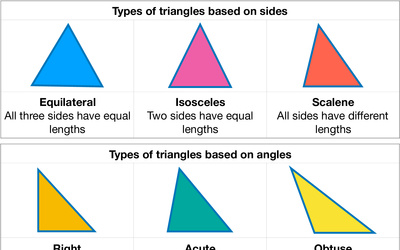# Number Blocks: First Ten Triangle NumbersTriangle numbers are a type of number sequence where each number in the sequence is the sum of all positive integers up to and including a certain number. For example, the first triangle number is 1, because it is the sum of all positive integers up to and including 1: 1 = 1.

Here are the first ten triangle numbers:
1
3 (1 + 2)
6 (1 + 2 + 3)
10 (1 + 2 + 3 + 4)
15 (1 + 2 + 3 + 4 + 5)
21 (1 + 2 + 3 + 4 + 5 + 6)
28 (1 + 2 + 3 + 4 + 5 + 6 + 7)
36 (1 + 2 + 3 + 4 + 5 + 6 + 7 + 8)
45 (1 + 2 + 3 + 4 + 5 + 6 + 7 + 8 + 9)
55 (1 + 2 + 3 + 4 + 5 + 6 + 7 + 8 + 9 + 10)

Each of these numbers is called a triangle number because you can represent them as a triangle of blocks. For example, the first triangle number (1) is just a single block. The second triangle number (3) can be represented as a triangle with one block at the top and two blocks on the next row, and so on.Different Types of Triangles: Equilateral, Isosceles, Scalene, Right, Acute, and Obtuse Triangles